# 「Number Talks」陪伴孩子建立數感，讓他們愛上數學

「Number Talks」的核心之一就是視覺化，孩子能在心裡思考來解答出答案

• 9 + (1 +3) = (9 + 1) + 3 = 10 + 3 = 13
• (3 + 6) + 4 = 3 + (6+4) = 3 + 10 = 13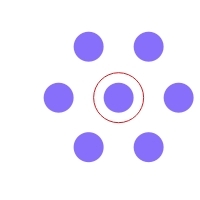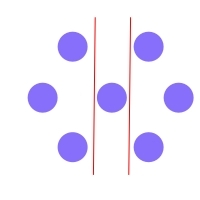1. 提問題：引導者將題目寫在白板上。

2. 請給小孩足夠的時間去獨自安靜地在腦中思考，不需要紙筆，也不需要和其他人討論。

3. 時間到了，引導者可以問問小孩們答案是多少？然後引導者將答案寫在白板上，並且也問問還有沒有其他答案，直到沒有其他答案為止。但在這時候，引導者是不需要將是誰回答的名字寫在答案旁邊。

4. 問問有沒有小孩志願分享解釋他的想法，若可以的話，甚至再進一步請他用「視覺化」來加強表示。引導者最好能鼓勵每位小孩都能分享他的思考策略，而小孩表達解釋他的思考策略時。並不是要小孩用以下的方式描述：15 x 8 答案是120，因為150 – 30。而是應該要類似這樣的方式回答，才能知道清楚描述思考脈絡。比如：因為數字湊成10比較好計算。而 8 = 10-2。所以15 x 10 – 15 x 2，這樣就比較容易計算。而小孩和我們就能清楚這小孩它採用了「以10為基準」的策略方式。

5. 小孩兩兩一組與旁邊的小孩一起討論，學會聆聽，學會一起思考

6. 記錄思考策略以及獲得到的啟發。

### 「Number Talks」有三個基本原則。

1. 數字加倍（特別用在加法與乘法）。這是在計算過程中，提醒將任意數字加倍的方式進行，如：6 + 6 或 6 x 2 。
• 題目範例：36加倍
• 參考策略：
• 我將36拆成30+6。而30 x 2 + 6 x 2 = 72
• 我知道35加倍是70，而1加倍是2，所以 70 + 2= 72

2. 數字減半（特別用在除法）。除法是平分的概念，如：30 = 15 + 15 或是 30 = 15 / 2
• 題目範例：36減半
• 參考策略：
• 我知道 36 = 30 + 6。我也知道15+15等於30，所以30減半是15，我也知道3+3等於6，所以 6減半是3，36減半是 15 + 3 = 18。
• 我知道 36 = 20 + 16。我也知道20除以2為10，我也知道16除以2等於8，所以36減半是 10 + 8 = 18。

3. 從十的次方開始。這個練習主要是幫助小孩日後遇到數字較大或較小時，也能輕鬆以對。
• 參考範例：36要加多少才能到100 (10的平方）
• 參考策略：
• 我要先湊成10的倍數這比較容易計算，所以36 + 4 = 40，而後再加 60 ，就等於100。因此答案是 4 + 60 = 64。
• 我知道100 是 60 + 40，而40和36差4。因此60 + 4 = 64。
• 我加一個十的倍數到最接近100，但不超過100，所以 36 + 60 = 96 ，然後 96 再加 4 就等於100。因此 60 + 4 = 64 。

## 四則運算的「Number Talks」思考策略

### 減法

1. 將減數四捨五入到十的倍數，然後調整。
• 37四捨五入到40。72 – 40 = 32 ，因我們四捨五入時拿走了3，因此得將3加回去，因此為 32+ 3 = 35
•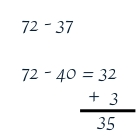2. 分解減數
•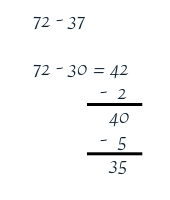3. 以加法替代，
•  以10的倍數為基準，用在被減數、減數或是用來透過10的倍數大幅縮短兩數字中間的距離。
• 方法一：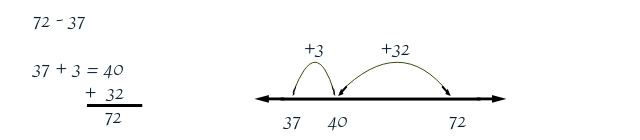• 方法二：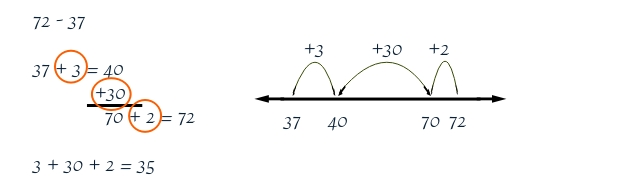• 方法三：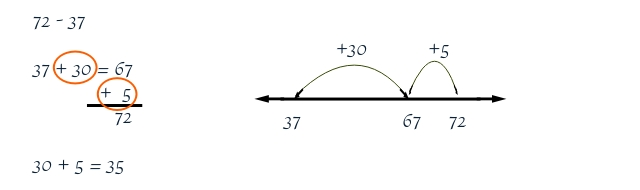4. 相同的差，平移。
•  這個策略非常實用且廣泛，採用的就是減法是距離的概念。而我個人也常用在方程式與代數上。
•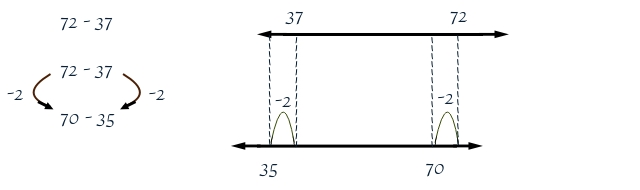5.  拆開分位
• 70 – 30 = 40，2 – 7 = -5。40 – 5 = 35
•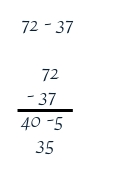### 乘法

1. 拆解乘數為兩個或多個加數
• 14 × ( 10 + 8 ) = ( 10 + 8 ) × 14  = 140 + 112 = 252

2. 拆解乘數為多個乘數。
• 而其中可採用加倍的方式一起運用，會比容易計算。而遇到乘數的個位數分別有 5 和 2時，也可以運用。
• 14 × ( 2 × 3 × 3 ) = 28 × 3 × 3 = 84 × 3 = 28 × 9 = 252
• 14 × 18 = (2 × 7 ) x ( 2 × 9 ) = 7 × 9 × 2 × 2 = 63 × 2 × 2 = 126 × 2 = 252
• 15 × 12 = (10 + 5 ) × 12 = 120 + 60 = 180

3. 四捨五入乘數，並作調整。
• 14 × ( 20 – 2 ) = 280 – 28 = 280 – (20 + 8 ) = 260 – 8 = 252

4. 減半和加倍
14 × 18 = 28 × 9 = 84 × 3 = 252

### 加法

1. 四捨五入後調整
• 54 + 27 = 54 + ( 30 – 3) = 84 – 3 = 81

2. 先取後給
•  這是一種分享的概念，也是一種等量平移的概念。
• 54 + 27 = ( 51 + 3 ) + 27 = 51 + ( 3+ 27) = 51 + 30 = 81
•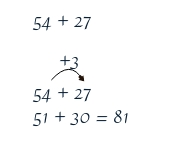3. 從左至右計算。
• 這做法與過去我們常用的由右至左的方式不同。我曾在一個印度的線上教學網站中，也看過他們鼓勵這樣的做法，而由左至右的方式，也不會失去了分位。我到現在還印象深刻，書上的費曼先生也是這麼計算著，他說這樣我就可以正確地「說出」答案了。
• 54 + 27 = (50 + 4) + (20 + 7) = (50 + 20) + (4 + 7) = 70 + 11= 81
•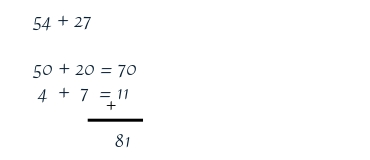4. 拆解一個加數
• 54 + 27 = 54 + (20 + 7) = 74 + 7 = 81
•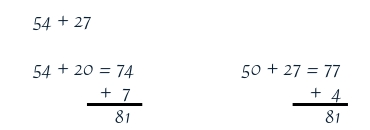5. 往上加。這和拆解一個加數很類似，但是更多的不同是採用視覺化來運算
•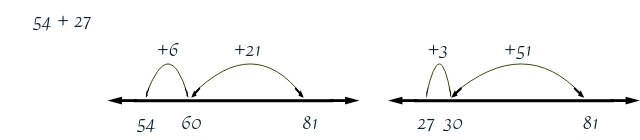### 除法

1. 以乘法替代
• 題目範例：17 ÷ 3
• 參考策略：因為我知道 5 × 3 = 15 ，所以我有五組3，因此我就知道答案是 商是5，餘是2。
2. 分塊
• 將數字視為一個一個大小不一的數字積木組合而成。你也可以想成是加法的「往上加」的策略。
• 題目範例： 739 ÷ 20
•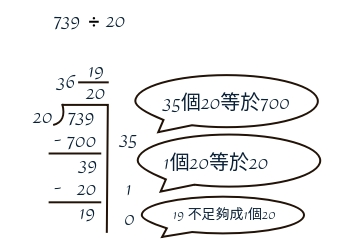3. 建一座數字塔
• 題目範例：688 ÷ 17
•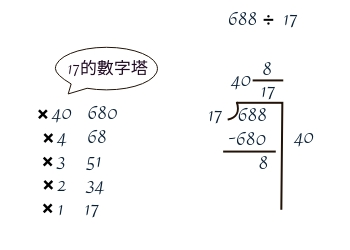4. 減半再減半
• 這是同時將被除數與除數一起減半的策略
• 題目範例：144 ÷ 36
•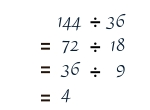「Number Talks」另一個特點「視覺化」，我就留到之後再說。